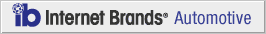How to Calculate Car Tax Depreciation

January 27, 2012

If you want to purchase a used car and are concerned about car tax, depreciation rates for a vehicle can help to provide an insight into how much you might expect to pay in sales or use taxes. The actual amount of sales or use tax you will be required to pay on any used vehicle will be determined by the final selling price.

Step 1 - Look up Original Manufacturer's Suggested Retail Price (MSRP)

In order to determine the depreciation rate for tax, you will first need to know the original MSRP of the vehicle you will be buying. Regardless if the vehicle is new or used, you will need to know this. One of the easiest ways to find the original MSRP for any vehicle is to visit the Kelley Blue Book Pricing Guide or the Edmunds Pricing guide and look up the make, model and trim type for the vehicle you are interested in.

Step 2 - How to Determine Car Residual Values

Determine the residual value of the vehicle. The residual value is actually a term when leasing a vehicle. The residual value is the amount a certain make and model is worth after a specified number of years. Sometimes, this amount is also referred to as the Net Capitalized Cost of the vehicle, as it also refers to how much a car depreciates or costs the owner to use over a specified period of time. In simpler terms, the residual value is a percentage of the MSRP that represents the vehicle's value at particular point in time.

Different banks and lenders use different formulas for calculating a car's residual value. However, the rule of thumb for this is that most vehicles depreciate at a rate of about 15% to 18% per year. If you use a value of 16.5% depreciation per year, you won't be very far off the mark. The residual value is the value of the vehicle after depreciation.

Step 3 - Compute Car Depreciation Value

Using our benchmark rate for depreciation of 16.5% per year, we can come up with a depreciation value and the vehicle's residual or approximate market value. Let's say that a new vehicle has an original MSRP of \$30,000 and is three years old.

If we use a depreciation rate of 16.5%, the vehicle depreciates and is valued as follows:

• After 1 Year - Depreciates \$4,950 and is valued at \$25,050
• After 2 Years - Depreciates another \$4,133.25 and is valued at \$20,916.75
• After 3 Years -  Depreciates another \$3,451.26 and is valued at \$17,465.49

As our do it yourself calculations show, the vehicle depreciates a total of \$12,534.51 in a period of just three years and the residual or final value is \$17,465.49. You should be aware that some vehicles do depreciate faster than others, and that this is not accurate for all vehicles. However, it is a good ballpark estimate.

Step 4 - Determine Depreciated Value Tax Amount

Since we now know the residual or average market value for our sample vehicle, we can now determine about how much sales or use tax we would need to pay for this vehicle. If you live in an area that charges 8% sales or use tax, you would calculate the sales tax using the following method:

Residual Value x Sales Tax Rate = Sales Tax Amount to be Paid

\$17,465.49 x 8% = \$1,397.24 estimated sales or use tax to be paid on a three year old used car with a residual value of \$17.465.49.

COPYRIGHT 1999-2019 MH Sub I, LLC dba CarsDirect.com